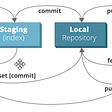# More patterns for coding interviews

Unpopular patterns that show up often in coding interviews

I assume you are already comfortable with common algorithms and data structure.
if you have not taken this course, you should start with it.

Examples are from Leetcode and solutions are in python

## 1- Traversing 2D space.

This pattern solves problems where you are given a 2D space or matrix and are required to traverse it in a given sequence of steps

This pattern is solved by defining position variables and a direction variable that indicates the next amount of shift in both x,y directions. then control the direction variable according to the problem statement

Turning left is reversing direction variable and multiplying x by -1
Turning right is reversing direction variable and multiplying y by -1
Moving forward is adding direction to the position

examples:

1041. Robot Bounded In Circle

`class Solution:    def isRobotBounded(self, instructions: str) -> bool:        x,y,direction = 0,0,(0,1)for instruction in instructions:            if instruction == 'G': x,y = x+direction,y+direction            elif instruction == 'L': direction = (-direction,direction)            else: direction = (direction,-direction)return (x==0 and y==0) or direction != (0,1)`

54. Spiral Matrix

`class Solution:    def spiralOrder(self, matrix: List[List[int]]) -> List[int]:        x, y, di, visited, ans = 0,0,(0,1),set(),[]for _ in range(len(matrix)*len(matrix)):            ans.append(matrix[x][y])            visited.add((x,y))                        if x+di in (-1,len(matrix)) or y+di in (-1, len(matrix)) or (x+di,y+di) in visited:                di = (di, - di)            x,y = x+di, y+di        return ans`

## 2- Unique combinations

This pattern solves problems where you need to generate subsets of a given sequence that meet a certain condition. but they need to be non-repeating nor a permutation of each other

for example [[1,1,2],[1,1,2],[1,2,1]] is not valid because 1st and 2nd are duplicates and all of them are permutations of each other

To avoid duplicate subsets, sort and skip neighboring elements with the same value
To avoid permutations in the sorted array, consider elements only larger than the current element. so in the previous example [1,2,1] won’t be possible to generate because 1 is less than 2 so it can’t come after it

examples:

15. 3Sum

`class Solution:    def threeSum(self, nums: List[int]) -> List[List[int]]:        if len(nums) < 3: return []        nums.sort()        res, seen_i = set(), {}                for i in range(len(nums)-2):            if i>0 and nums[i]==nums[i-1]: continue            seen_k = {}            for j in range(i+1,len(nums)):                k = -nums[i]-nums[j]                if nums[j] in seen_k: res.add((nums[i], k, nums[j]))                else: seen_k[k]=1        return map(list, res)`

40. Combination Sum II

`class Solution:    def combinationSum2(self, candidates: List[int], target: int) -> List[List[int]]:        ans = []        candidates.sort()        def dfs(candidates,route,target):            if target==0: return ans.append(route)            for i, candidate in enumerate(candidates):                if candidate>target: break                if i>0 and candidate==candidates[i-1]: continue                dfs(candidates[i+1:], route+[candidate], target-candidate)        dfs(candidates,[],target)        return ans`

## 3- Monotonic subarray

This pattern solved sorted and shifted array problems.
given a sorted, shifted array. one half of it is monotonic and the other one is not. you can use this property to eliminate one half at each step

Assuming the array is in increasing order, you can find the monotonic half by comparing the middle element with the last one, if the latter is larger then the right half is monotonic. else, the left one is.

examples:

33. Search in Rotated Sorted Array

`class Solution:    def search(self, nums: List[int], target: int) -> int:        if not nums: return -1left, right = 0, len(nums) - 1while left <= right:            mid = (left + right) // 2            if target == nums[mid]: return mid            if nums[mid]>=nums[left]:                if nums[left]<=target<=nums[mid]: right= mid-1                else: left = mid+1            else:                if nums[mid]<=target<=nums[right]: left = mid+1                else: right= mid-1return -1`

154. Find Minimum in Rotated Sorted Array II

`class Solution:    def findMin(self, nums: List[int]) -> int:        lo, hi = 0,len(nums)-1        while lo<hi:            mid = (lo+hi)//2            if nums[hi]>=nums[mid]: hi=mid if nums[hi] != nums[mid] else hi - 1            else:lo=mid+1        return nums[lo]`

It’s getting too long. so I’m going to end it here
Give a clap if you are interested in more patterns :)

UPDATE: Part 2 and Part 3 are published

--

--

--

## More from Muhammed Abogazia

human

Love podcasts or audiobooks? Learn on the go with our new app.

## A bright future for Frame## Transformers in Swift## Most Frequently Used Git Commands## Using platform variants when testing flutter widgets## Build and deploy a Maven project with circleci to herokuhuman

## Bee Travel Puzzle## Steps to design a Dynamic programming algorithm